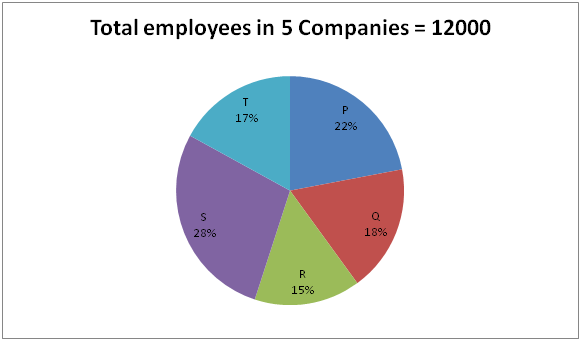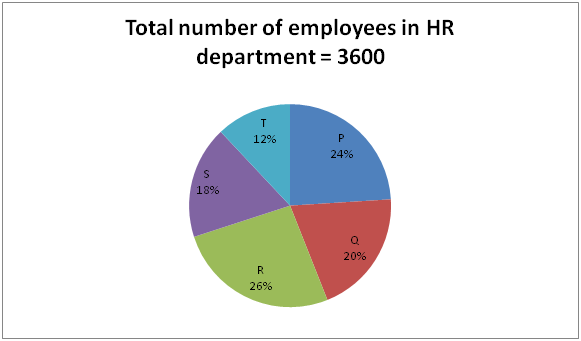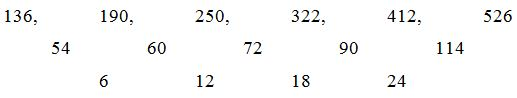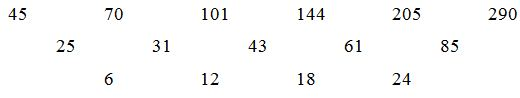# SBI PO Prelims Quantitative Aptitude Questions 2019 (Day-45)

Dear Aspirants, Our IBPS Guide team is providing new series of Quantitative Aptitude Questions for SBI PO 2019 so the aspirants can practice it on a daily basis. These questions are framed by our skilled experts after understanding your needs thoroughly. Aspirants can practice these new series questions daily to familiarize with the exact exam pattern and make your preparation effective.

[WpProQuiz 6348]

### Click Here for SBI PO Pre 2019 High-Quality Mocks Exactly on SBI Standard

Click Here to View Quantitative Aptitude Questions in Hindi

Directions (Q. 1 – 2): Each question given below contains a statement followed by Quantity I and Quantity II. Find the relationship between them and mark your answer as,

a) Quantity I > Quantity II
b) Quantity I ≥ Quantity II
c) Quantity II > Quantity I
d) Quantity II ≥ Quantity I
e) Quantity I = Quantity II or Relation cannot be established

1) Quantity I: The perimeter of rectangle is 172 cm and the difference between the length and breadth of the rectangle is 14 cm. Find the area of the rectangle?

Quantity II: 2400 Sq cm

2) Quantity I: A and B started a business by investing in the ratio of 5: 8. If 20% of the total profit goes to Charity and the remaining profit will be shared by both of them and A’s share is Rs. 20000, then find the total profit?

Quantity II: Rs. 50000

Directions (Q. 3 – 5): The questions given below are based on the given Series-I. The series-I satisfy a certain pattern, follow the same pattern in Series-II and answer the questions given below.

3) I) 24, 12, 18, 45, 157.5

II) 56 ……. 367.5. If 367.5 is nth term, then what value should come in place of (n + 1)th term?

a) 1827.25

b) 1653.75

c) 2068.75

d) 1946.25

e) None of these

4) I) 5, 11, 34, 171, 1198

II) 13 ……. 31659. If 31659 is nth term, then find the value of n?

a) 4

b) 7

c) 5

d) 6

e) None of these

5) I) 136, 190, 250, 322, 412, 526

II) 45, 70 ……. 290. If 290 is nth term, then what value should come in place of (n – 2)th term?

a) 144

b) 156

c) 188

d) 172

e) None of these

Directions (Q. 6 – 10): Study the following information carefully and answer the given questions.

The following pie chart1 shows the percentage distribution of total number of employees working in 4 different departments of five different companies.The pie chart2 shows the percentage distribution of total number of employees working in HR department of five different companies.6) Find the difference between the total number of employees working in HR department in company P to that of total number of employees  working other than HR department in company S?

a) 1848

b) 1562

c) 1046

d) 2154

e) None of these

7) Find the ratio between the total number of employees working other than HR department in company Q to that of total number of employees working in 4 departments in company R?

a) 7 : 9

b) 11 : 13

c) 25 : 36

d) 4 : 5

e) None of these

8) Find the average number of employees working in 4 departments of company P, R and T together?

a) 2340

b) 2160

c) 2580

d) 1920

e) None of these

9) If the ratio between the male to that of female employees working in HR department of company R and S is 13 : 11 and 7 : 5 respectively, then find the total number of male employees working in HR department in company R and female employees working in company S together?

a) 828

b) 916

c) 777

d) 1035

e) None of these

10) Total number of employees working other than HR department in company T is approximately what percentage of total number of employees working in HR department of same company?

a) 372 %

b) 336 %

c) 290 %

d) 408 %

e) 445 %

Answers :

1) Answer: c)

Quantity I:

The perimeter of rectangle = 172 cm

2*(l + b) = 172

l + b = 86 –> (1)

The difference between the length and breadth of the rectangle = 14 cm

l – b = 14 —> (2)

By solving the equation (1) and (2), we get,

Length = 50 cm, Breadth = 36 cm

The area of the rectangle = l*b = 50*36 = 1800 cm^2

Quantity II:

2400 cm^2

Quantity II > Quantity I

2) Answer: a)

Quantity I:

The investment ratio of A and B = 5 : 8

Here, the period is not given. So,

The ratio of investment = The ratio of profit

A’s share = Rs. 20000

5’s = 20000

1’s = 4000

13’s = 52000

80 % of total profit = 52000

Total profit = 52000*(100/80) = Rs. 65000

Quantity II:

Rs. 50000

Quantity I > Quantity II

Direction (3-5) :

3) Answer: b)

Series I pattern:

24*0.5 = 12

12*1.5 = 18

18*2.5 = 45

45*3.5 = 157.5

Series II pattern:

56*0.5 = 28

28*1.5 = 42

42*2.5 = 105

105*3.5 = 367.5 (nth term)

367.5*4.5 = 1653.75 [(n + 1)th term]

4) Answer: d)

Series I pattern:

5*2 + 1 = 11

11*3 + 1 = 34

34*5 + 1 = 171

171*7 + 1 = 1198

1198*11 + 1 = 13179

Series II pattern:

13 is the first term.

13*2 + 1 = 27

27*3 + 1 = 82

82*5 + 1 = 411

411*7 + 1 = 2878

2878*11 + 1 = 31659

31659 is 6th term. So, n = 6

5) Answer: a)

Series I pattern:Series II pattern:

45 is first term.nth term = 290

(n – 2)th term = 144

Directions (Q. 6 – 10):

6) Answer: a)

The total number of employees working in HR department in company P

= >3600*(24/100) = 864

The total number of employees working other than HR department in company S

= >[12000*(28/100)] – [3600*(18/100)]

= > 3360 – 648 = 2712

Required difference = 2712 – 864 = 1848

7) Answer: d)

The total number of employees working other than HR department in company Q

= >[12000*(18/100)] – [3600*(20/100)]

= > 2160 – 720 = 1440

The total number of employees working in 4 departments in company R

= >12000*(15/100) = 1800

Required ratio = 1440 : 1800 = 4 : 5

8) Answer: b)

The average number of employees working in 4 departments of company P, R and T together

= >[12000 * (22 + 15 + 17)/100]/3

= > 2160

9) Answer: c)

The total number of male employees working in HR department in company R

= >3600*(26/100)*(13/24) = 507

The total number of female employees working in HR department in company S

= >3600*(18/100)*(5/12) = 270

Required sum = 507 + 270 = 777

10) Answer: a)

Total number of employees working other than HR department in company T

= >[12000*(17/100)] – [3600*(12/100)]

= > 2040 – 432 = 1608

Total number of employees working in HR department of company T

= >3600*(12/100) = 432

Required % = (1608/432)*100 = 372.22 % = 372 %

Related Articles :

SBI PO Prelims Quantitative Aptitude Questions 2019 (Day-44)

0 0 votes
Rating0 Comments
Inline Feedbacks
View all comments